color codes currently in use are the Joint Army-Navy (JAN) code and the Radio Manufacturers' Association (RMA) code. ">Custom SearchCOLOR CODES FOR CAPACITORS

Although the capacitance value may be printed on the body of a capacitor, it may also be indicated by a color code. The color code used to represent capacitance values is similar to that used to represent resistance values. The color codes currently in use are the Joint Army-Navy (JAN) code and the Radio Manufacturers' Association (RMA) code.

For each of these codes, colored dots or bands are used to indicate the value of the capacitor. A mica capacitor, it should be noted, may be marked with either three dots or six dots. Both the three- and the six-dot codes are similar, but the six-dot code contains more information about electrical ratings of the capacitor, such as working voltage and temperature coefficient.

The capacitor shown in figure 3-21 represents either a mica capacitor or a molded paper capacitor. To determine the type and value of the capacitor, hold the capacitor so that the three arrows point left to right (>). The first dot at the base of the arrow sequence (the left-most dot) represents the capacitor TYPE. This dot is either black, white, silver, or the same color as the capacitor body. Mica is represented by a black or white dot and paper by a silver dot or dot having the same color as the body of the capacitor. The two dots to the immediate right of the type dot indicate the first and second digits of the capacitance value. The dot at the bottom right represents the multiplier to be used. The multiplier represents picofarads. The dot in the bottom center indicates the tolerance value of the capacitor.

Figure 3-21. - 6-dot color code for mica and molded paper capacitors.Example of mica capacitors.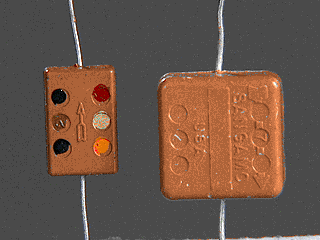Example of mica capacitors.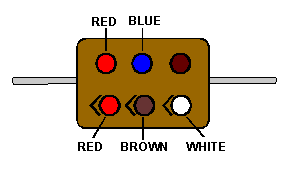To read the capacitor color code on the above capacitor:

Hold the capacitor so the arrows point left to right.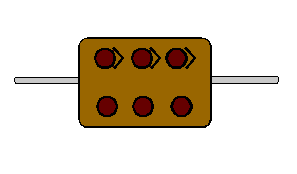Read the first dot.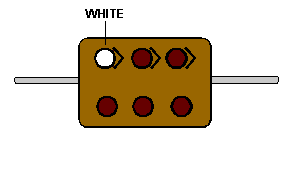Read the first digit dot.Read the second digit dot and apply it to the first digit.Read the multiplier dot and multiply the first two digits by multiplier (Remember that the multiplier is in picofarads).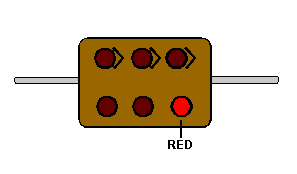Lastly, read the tolerance dot.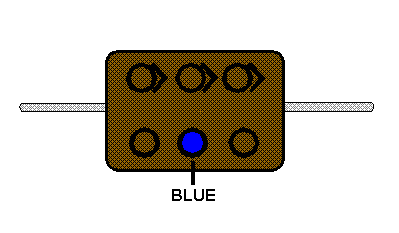According to the above coding, the capacitor is a mica capacitor whose capacitance is 1200 pF with a tolerance of ±6%.

The capacitor shown in figure 3-22 is a tubular capacitor. Because this type of capacitor always has a paper dielectric, the type code is omitted. To read the code, hold the capacitor so the band closest to the end is on the left side; then read left to right. The last two bands (the fifth and sixth bands from the left) represent the voltage rating of the capacitor. This means that if a capacitor is coded red, red, red, yellow, yellow, yellow, it has the following digit values:

 red=2 red=2 red=X 100 pF yellow=±40% yellow=4 yellow=4

Figure 3-22. - 6-band color code for tubular paper dielectric capacitors.

<figureNE0178">

The six digits indicate a capacitance of 2200 pF with a ±40 percent tolerance and a working voltage of 44 volts.

The ceramic capacitor is color coded as shown in figure 3-23 and the mica capacitor as shown in figure 3-24.

Notice that this type of mica capacitor differs from the one shown in figure 3-21 in that the arrow is solid instead of broken. This type of mica capacitor is read in the same manner as the one shown in figure 3-21, with one exception: the first dot indicates the first digit. (Note: Because this type of capacitor is always mica, there is no need for a type dot.)

Figure 3-23. - Ceramic capacitor color code.Figure 3-24. - Mica capacitor color code.Q.19 Examine the three capacitors shown below. What is the capacitance of each?Privacy Statement - Copyright Information. - Contact Us